# Evaluate Formula

This allows you to break up the formulas into smaller pieces.
Press Step In to separate a formula into smaller chunks
This feature was introduced in Excel 2002.
Formulas can quickly become very complicated, especially if you are using nested worksheet functions.
This allows you to break up your long formulas into smaller ones and to evaluate them separately.
Very useful when you have cell references and nested worksheet functions.
This helps you debug complex formulas by stepping you through each calculation and displaying the results.
This dialog box displays the formula and the values that are supplied as arguments.
If your formula does not return the correct value then you can use the Evaluate Formula dialog box to step into the formula.
It does not allow you to change the formula only to step though it. If you want to change the formula you must close the Evaluate Formula dialog box.

You can use the F9 key to evaluate parts of your formulas. Highlight the portion of the formula that you want to resolve and press the F9 key.
Always press the ESC key afterwards. Be careful not to press the Enter key as this will result in your formula being permanently changed. This can be used to see the values that a range is actually returning.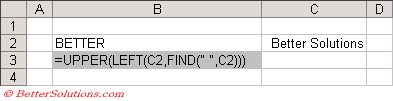The formula in cell B2 contains a cell reference as well as two nested worksheet functions.
The actual formula "=UPPER(LEFT(C2,FIND(" ", C2)))" is displayed in cell B3.
This is a relatively simple formula compared to the formulas that you could create.

This formula can be broken up into individual steps:
1) The FIND function is used to locate the character position of the first space in the text "Better Solutions".
2) The LEFT function is then used to obtain all the text that is to the left of this space. In this case the first word "Better".
3) The UPPER function is then used to convert all the characters to uppercase resulting in the final value which is the text "BETTER".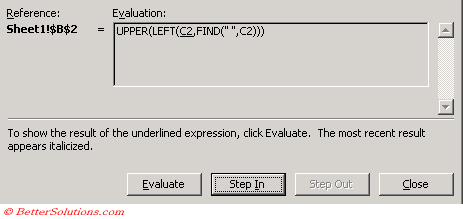Evaluate - Replaces any calculated arguments with their actual values. Shows result of an underlined expression or the value represented by an underlined cell reference.
Step In - Allows you to examine the formula represented by the underlined cell reference. This is not available when the cell being referenced is in another workbook. Allows you to view the actual contents of any referenced cells before applying it to the formula by displaying it in a separate box.
Step Out - Applies the argument to the function and combines the process.

Selecting "Step In" evaluates the cell C2.
This separates that particular piece of the formula and evaluates it separately in the box underneath.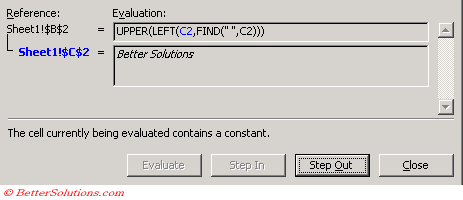Selecting "Step Out" will substitute the cell reference for the actual value in the formula.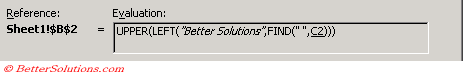Every time you press "Evaluate" another nested level of the formula will be evaluated and substituted.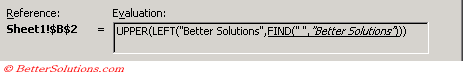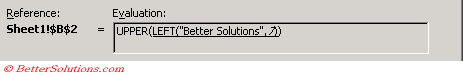Eventually the Evaluation box will display the actual result of the formula and the "Evaluate" button will change to a "Restart" button allowing you to step through the formula again.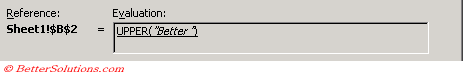When all arguments have been calculated, the final result of the formula is displayed.
You can press the "Restart" button to step through the formula again.

### Important

You can click the Evaluate button as many times as necessary to replace all the calculated arguments in your nested formulas
It is possible to obtain a graphical representation of your formulas by adding trace arrows to your worksheet.